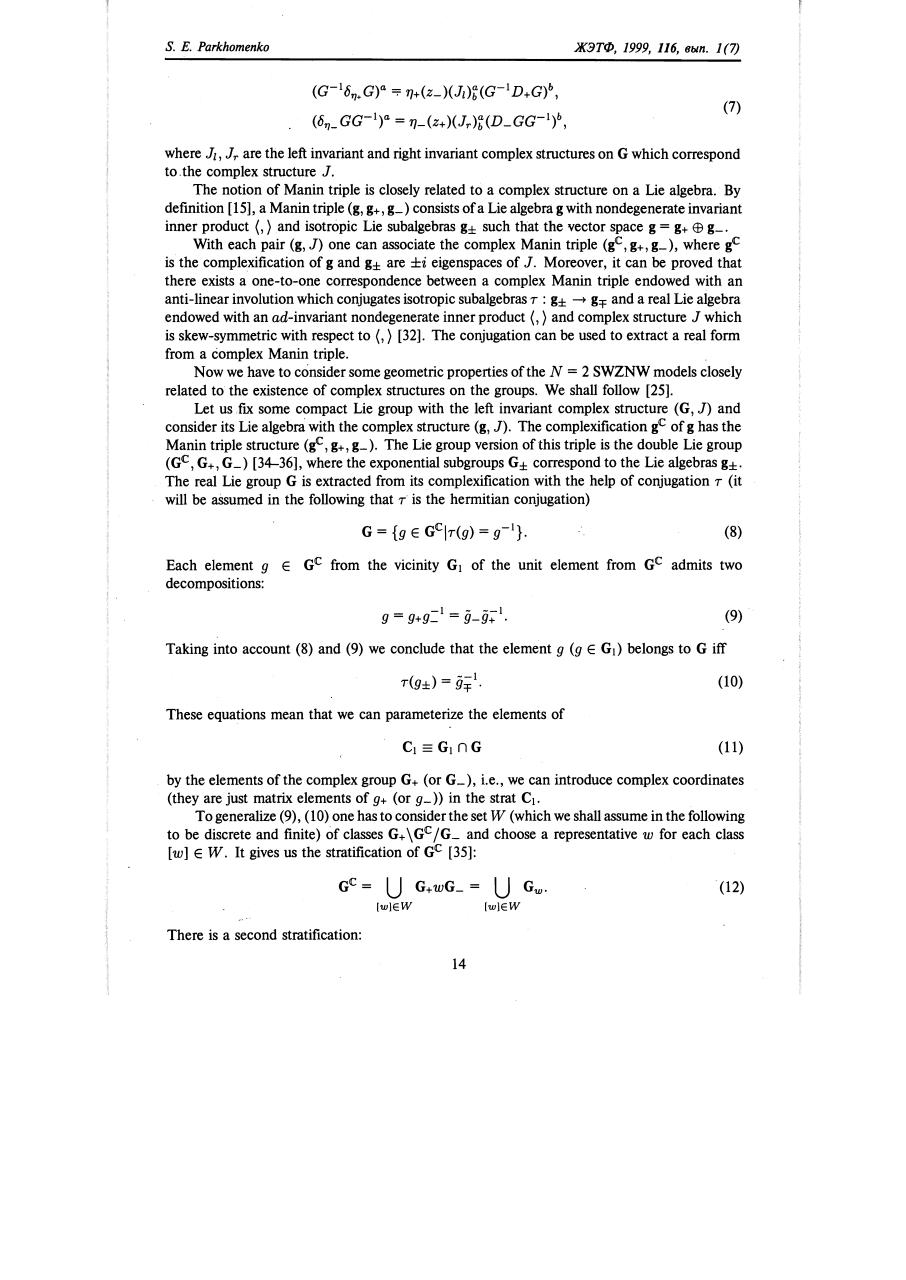# r 116 0011.pdfPage 1 2 3 45615

#### Text preview

s.

Е.

Parkhomenko

ЖЭТФ,

1999, 116,

вьtn.

1 (7)

(G-1Оry+G)а &quot;&quot; 1}+(z_)(J1 )'t(G- 1 D+G)Ь,
(Ory_GG-1)а

(7)

= 1}_(Z+)(Jr)'t(D_GG- 1 )Ь,

where J1, Jr are the left invariant and right invariant complex structures оп G which couespond
to.the complex structure J.
The notion of Manin triple is closely related to а complex structure оп а Lie algebra. Ву
definition , а Manin triple (g, g+, g_) consists of а Lie algebra g with nondegenerate invariant
inner product (,) and isotropic Lie subalgebras g± such that the vector space g = g+ ЕВ g_.
With each pair (g, J) one сап associate the complex Manin triple (glC, g+, g_ ), where glC
is the complexification of g and g± are ±i eigenspaces of J. Moreover, it сап Ье proved that
there exists а one-to-one couespondence between а complex Manin triple endowed with an
anti-linear involution which conjugates isotropic subalgebras r : g± -+ g'f and а real Lie algebra
endowed with an ad-invariant nondegenerate inner product (,) and complex structure J which
is skew-symmetric with respect to (,) . The conjugation сап Ье used to extract а real [оun
from а complex Manin triple.
Now we have to consider some geometric properties ofthe N = 2 SWZNW models closely
related to the existence of complex structures оп the groups. We shall follow .
Let us ТlX some compact Lie group with the left invariant complex structure (G, J) and
consider its Lie algebrn with the complex structure (g, J). Тhe complexification glC of g has the
Manin triple structure (glC, g+, g_ ). The Lie group version of this triple is the double Lie group
(GIC, G+, G_) [34-36], where the exponential subgroups G± couespond to the Lie algebras g±.
The real Lie group G is extracted from its complexification with the help of conjugation r (it
wi1l Ье assumed in the following that r is the heunitian conjugation)
(8)
Each element 9
decompositions:

Е

GIC from the vicinity G 1 of the unit element from GIC admits two

(9)

Taking into account (8) and (9) we conclude that the element 9 (9

Е

G 1) belongs to G iff
(10)

These equations mean that we

сап

parameterize the elements of
(11)

Ьу

the elements ofthe complex group G+ (or G_), i.e., we сап introduce complex coordinates
(they are just matrix elements of 9+ (or 9_» in the strat C 1 •
То generalize (9), (10) one has to consider the set W (which we shall assume in the following
to Ье discrete and finite) of classes G+ \ GIC /G_ and choose а representative w for each class
[w] Е W. It gives us the stratification of GIC :
. (12)
[w]EW

There is

а

[w]EW

second stratification:
14